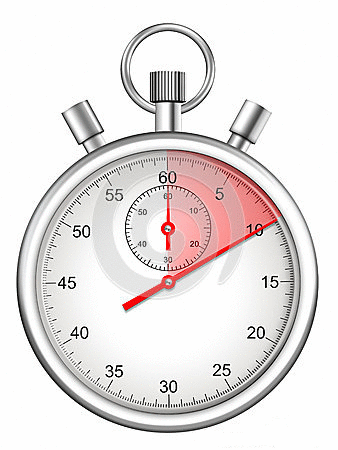top of page
Search
• Andrew Jones

# Timing your code in R

Having code that runs without failing is good enough most of the time! However, on occasion you may want to know how long a certain piece of code takes to run, this may be for efficiency purposes or to estimate run-times in a scaled up scenario.

In R there is a primitive function which helps you do just this! This function is proc.time() and is used like this:

ptm <- proc.time()

proc.time() - ptm

It records the time as the code commences and saves this to object 'ptm', then subtracts this from the time recorded when the code finishes - giving the difference between the two. To illustrate this in practice, we'll use a snippet of code that we ran in our post about estimating Pi using Monte Carlo simulation.

The code in question iteratively looped through a doubling number of dart throws from 2^2 to 2^25. It isn't the most efficient code but it''ll do nicely for this example! We'll add in the proc.time() functionality to show it in action and to also discuss the results:

# create empty data frame to append estimations to pi_estimation_final <- data.frame(darts_thrown=numeric(0),pi_estimate=numeric(0))

#loop through varying numbers of darts, in this case we'll start with 2^2 darts (4) and keep doubling this until 2^25 darts (33.5 million darts) ptm <- proc.time() for (i in 2^(2:25)){ throws = i # draw random numbers for the x and y co-ordinates of the darts x_coordinate <- runif(throws,0,1) y_coordinate <- runif(throws,0,1) # use the pythagorean theorem dart_landing_point <- sqrt((x_coordinate^2) + (y_coordinate^2)) # count the number of darts that landed within the circle darts_inside_circle <- sum(dart_landing_point < 1) # estimate pi for this iteration pi_estimation <- data.frame(darts_thrown = throws, pi_estimation = (darts_inside_circle/throws)*4) # append this iteration to our final table pi_estimation_final <- rbind(pi_estimation_final,pi_estimation) } proc.time() - ptm

The result from this is as follows:

user system elapsed 6.22 0.73 6.96

The print-out of proc.time() shows three elements, the first two are the total user and system CPU times (seconds) of the process, and the third entry, elapsed, is the real elapsed time between the process starting and ending.

Hopefully you'll put this to good use! Please share using the buttons below